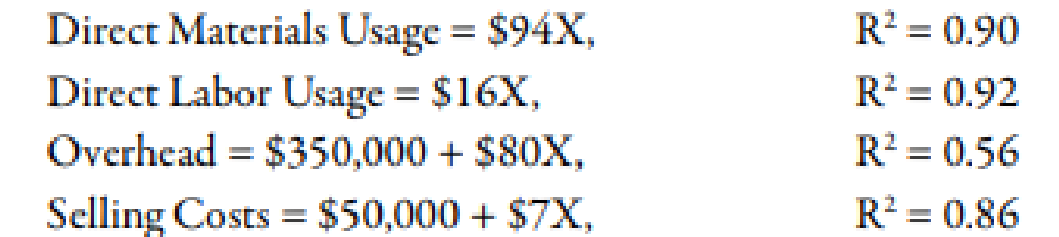Chapter 3, Problem 70C### Managerial Accounting: The Corners...

7th Edition
Maryanne M. Mowen + 2 others
ISBN: 9781337115773

#### Solutions

Chapter
Section### Managerial Accounting: The Corners...

7th Edition
Maryanne M. Mowen + 2 others
ISBN: 9781337115773
Textbook Problem
1 views

# (Appendix 3A) Cost Formulas, Single and Multiple Cost DriversFor the past 5 years, Garner Company has had a policy of producing to meet customer demand. As a result, finished goods inventory is minimal, and for the most part, units produced equal units sold.Recently, Garner’s industry entered a recession, and the company is producing well below capacity (and expects to continue doing so for the coming year). The president is willing to accept orders that at least cover its variable costs so that the company can keep its employees and avoid layoffs. Also, any orders above variable costs will increase overall profitability of the company. Toward that end, the president of Garner Company implemented a policy that any special orders will be accepted if they cover the costs that the orders cause.To help implement the policy, Garner’s controller developed the following cost formulas:where X = direct labor hours.Required: 1. Compute the total unit variable cost. Suppose that Garner has an opportunity to accept an order for 20,000 units at $212 per unit. Each unit uses 1 direct labor hour for production. Should Garner accept the order? (The order would not displace any of Garner’s regular orders.) 2. (Appendix 3A) Explain the significance of the coefficient of determination measures for the cost formulas. Did these measures have a bearing on your answer in Requirement 1? Should they have a bearing? Why? 3. (Appendix 3A) Suppose that a multiple regression equation is developed for overhead costs: Y =$100,000 + $85X1 +$5,000X2 + $300X3, where X1 = Direct Labor Hours, X2 = Number of Setups, and X3 = Engineering Hours. The coefficient of determination for the equation is 0.89. Assume that the order of 20,000 units requires 12 setups and 600 engineering hours. Given this new information, should the company accept the special order referred to in Requirement 1? Is there any other information about cost behavior that you would like to have? Explain. 1. To determine Calculate the amount of total unit variable cost. Also, identify whether the GA accept the order or not. Explanation Cost: Cost can be defined as the cash and cash equivalent which is incurred against the products or its related services which will benefit the organization in the future. There are two types of costs that are fixed and variable costs. Calculation of total unit variable cost:  Particulars Cost (A) ($) Units (B) Amount (A/B) (\$) Materials 94 20,000 1,880,000 Labor 16 20,000 320,000 Variable overhead 80 20,000 1,600,000 Variable selling 7 20,000 140,000 Total cost 3,940,000

2.

To determine

Discuss the importance of the coefficient of the determination in calculating the cost formula. Also, identify whether the cost formula is used in part 1 or not and give reason.

3.

To determine

Identify whether the company accept the special order which is referred in requirement 1. Also, identify whether the company needs any additional information about the cost behavior.

### Still sussing out bartleby?

Check out a sample textbook solution.

See a sample solution

#### The Solution to Your Study Problems

Bartleby provides explanations to thousands of textbook problems written by our experts, many with advanced degrees!

Get Started

#### Find more solutions based on key concepts